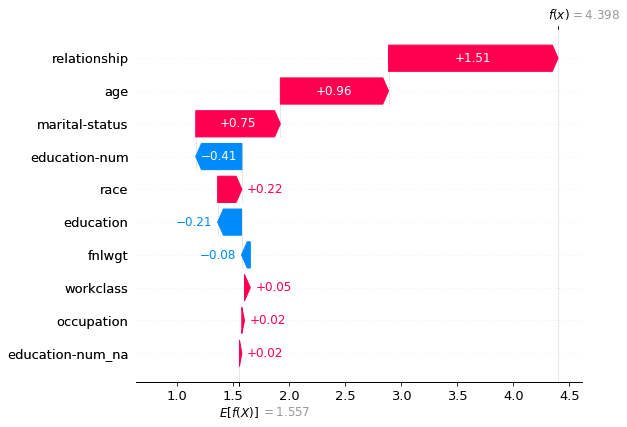## Lesson Video:

This article is also a Jupyter Notebook available to be run from the top down. There will be code snippets that you can then run in any environment.

Below are the versions of fastai, fastcore, wwf, and fastinference currently running at the time of writing this:

• fastai: 2.1.10
• fastcore: 1.3.13
• wwf: 0.0.8
• fastinference: 0.0.35

SHAP is a library for interpreting neural networks, and we can use it to help us with tabular data too! I wrote a library called FastSHAP which ports over the usabilities of it. Let's do a walkthrough of what each does and how it works.

• Note: I only have it ported for tabular data

## How to use it

FIrst we need to train a model. We'll quickly train our ADULTS model now:

from fastai.tabular.all import *

path = untar_data(URLs.ADULT_SAMPLE)

dep_var = 'salary'
cat_names = ['workclass', 'education', 'marital-status', 'occupation', 'relationship', 'race']
cont_names = ['age', 'fnlwgt', 'education-num']
procs = [Categorify, FillMissing, Normalize]

splits = IndexSplitter(list(range(800,1000)))(range_of(df))
to = TabularPandas(df, procs, cat_names, cont_names, y_names="salary", splits=splits)

learn = tabular_learner(dls, layers=[200,100], metrics=accuracy)
learn.fit(1, 1e-2)

epoch train_loss valid_loss accuracy time
0 0.361666 0.381548 0.835000 00:05

Now let's go through some example usage!

## fastinference

First let's import the interpretability module:

from fastinference.tabular import *


And now we'll make a ShapInterpretation object. It expects your Learner along with some test data to look at and any keywords that SHAP can use. If you don't pass anything in it will use a subset of your validation data:

exp = ShapInterpretation(learn, df.iloc[:100])


Let's look at the various methods available to us:

## Decision Plot

The decisio plot will visualize a model's decision by looking at the "SHAP" values for a particular row. If you plot too many samples at once it can make your plot illegible.

Let's look at the tenth row of our dataframe:

df.iloc

age                           23
workclass                Private
fnlwgt                    529223
education              Bachelors
education-num                 13
marital-status     Never-married
occupation                   NaN
relationship           Own-child
race                       Black
sex                         Male
capital-gain                   0
capital-loss                   0
hours-per-week                10
native-country     United-States
salary                      <50k
Name: 10, dtype: object

As we can see, our y value is '<50k'. Let's look at how the model performed and what could have been influencing our result into an opposite direction

exp.decision_plot(class_id=0, row_idx=10)

Classification model detected, displaying score for the class <50k.
(use class_id to specify another class)
Displaying row 10 of 100 (use row_idx to specify another row)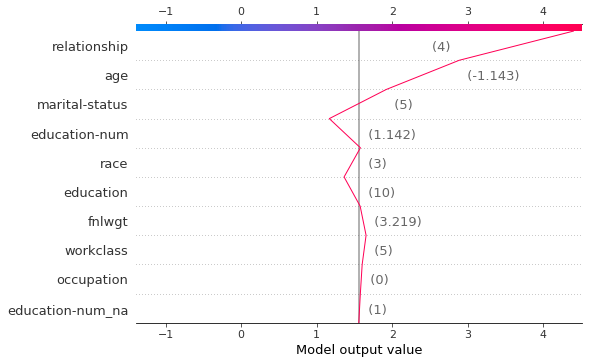And now if we turn this the other way around:

exp.decision_plot(class_id=1, row_idx=10)

Classification model detected, displaying score for the class >=50k.
(use class_id to specify another class)
Displaying row 10 of 100 (use row_idx to specify another row)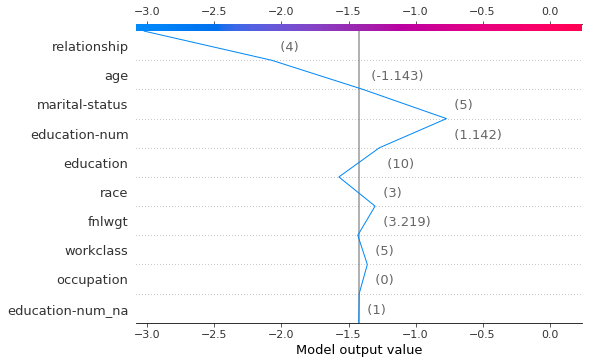We can visually see what variables were having the largest impact on the model. (Note, it shows the pre-processed datapoints)

## Dependency Plots

Dependency plots use the same variable on the x and y axis, with the y axis being the "SHAP" values of it. We can pass in a variable name and a particular class ID and it will show the dependency plot for all of the test data we passed in:

exp.dependence_plot('age', class_id=0)

Classification model detected, displaying score for the class <50k.
(use class_id to specify another class)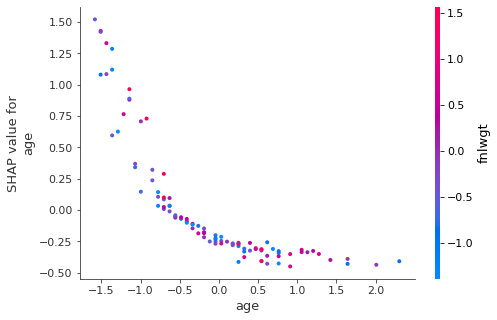exp.dependence_plot('age', class_id=1)

Classification model detected, displaying score for the class >=50k.
(use class_id to specify another class)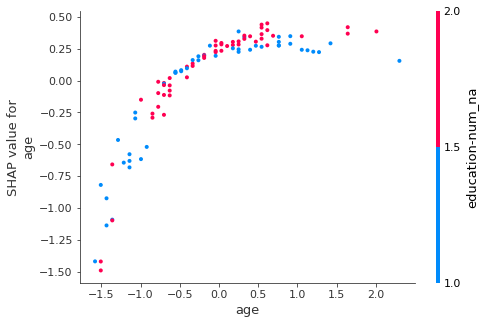## Force Plot

Fore plots will visualize the "SHAP" values with an added force layout. We can see how each variable at a certain value affects whether it falls into class A or class B:

exp.force_plot(class_id=1)

Classification model detected, displaying score for the class >=50k.
(use class_id to specify another class)Visualization omitted, Javascript library not loaded!
Have you run initjs() in this notebook? If this notebook was from another user you must also trust this notebook (File -> Trust notebook). If you are viewing this notebook on github the Javascript has been stripped for security. If you are using JupyterLab this error is because a JupyterLab extension has not yet been written.

## Summary Plot

Similar to feature importance, it shows the average impoact of a particular value on model performance:

exp.summary_plot()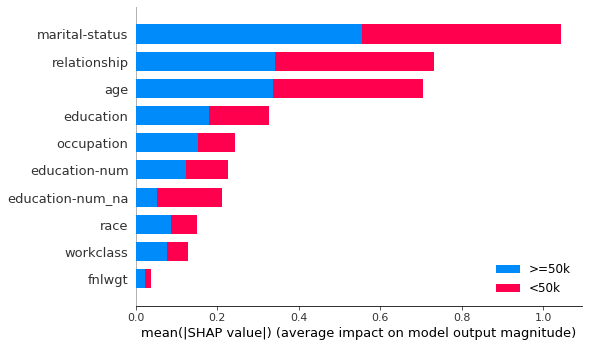## Waterfall Plot

And finally the waterfall plot. It'll explain a single prediction. It can accept a row_index and a class_id which defualts to the first one. It can be an integer or string representation of the class we want to look at. Let's look at that row 10 again:

exp.waterfall_plot(row_idx=10, class_id='<50k')

Classification model detected, displaying score for the class <50k.
(use class_id to specify another class)
Displaying row 10 of 100 (use row_idx to specify another row)